•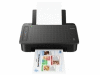• 更多图片

￥358•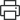产品定位：照片打印机
•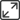最大打印幅面：A4
•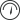黑白打印速度(ppm)：文档，ESAT/单面：黑白：7.7ipm，彩色：4ipm 照片(4"×6"，PP-201/无边距)：65秒
•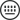网络功能：支持无线网络打印
•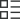进纸容量：后部手动进纸托盘(最大数量)： 普通纸：A4，LTR=60 高级光面照片纸II(PP-201)：4"×6"=20 光面照片纸(GP-508)：4"×6"=20 高级光面照片纸(PP-208)：4"×6"=20 照片贴纸(PS-108)：4"×6"=1 照片贴纸(PS-208)：4"×6"=1 照片贴纸(PS-308R)：4"×6"=1 照片贴纸(PS-808)：4"×6"=1 磁性照片纸(PS-508)：4"×6"=1 佳能奥西红色标签纸(WOP111)：A4=60 佳能奥西办公色彩纸(SAT213)：A4=50
A4喷打排行
500元以下喷打排行